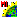# VTOOLKIT.sml

###Download

#### Syntax Highlighing:

```comments, key words, predefined symbols, class members & methods, functions & classes
```
```# VTOOLKIT.SML
# sample script for tutorial "Writing Scripts with SML.
# illustrating use of vector toolkit.
# Use information from an input raster to add elements to a new vector object.
# revised and updated 5 November 2009
clear();
# Get input raster and find its geographic extents in map
### coordinates defined by its georeference object.
class RVC_RASTER R;
GetInputRaster(R);
# get georeference and coordinate reference system for input raster
class RVC_GEOREFERENCE rGeoref;
R.GetDefaultGeoref(rGeoref);
printf("Raster CRS = %s\n", rGeoref.GetCoordRefSys().Name);
# get extents of the input raster in object (line and column) coordinates
class RECT3D extents;
R.GetExtents(extents);
# get coordinate transformation from raster object to map coordinates from the georeference
class TRANS2D_MAPGEN rTransObjToMap;
rGeoref.GetTransParm(rTransObjToMap, 0, rGeoref.GetCalibModel() );

# convert raster extents RECT3D to map coordinates
extents = rTransObjToMap.ConvertRectFwd(extents);
printf("Minimum extents of raster: MinX = %.2f, MinY = %.2f\n", extents.x1, extents.y1);
printf("Maximum extents of raster: MaxX = %.2f, MaxY = %.2f\n", extents.X2, extents.y2);
# Get maximum raster value and find line,column
# position of maximum. (Stop search at first instance.)
numeric rmax, lin, col, maxline, maxcol, max_X, max_Y;
rmax = GlobalMax(R);
for each R[lin,col] {
if (R == rmax) {
maxline = lin;
maxcol = col;
break;
}
}
printf("Maximum raster value = %d\n", rmax);
printf("Line number of maximum value = %d\n", maxline);
printf("Column number of maximum value = %d\n", maxcol);
# Convert max cell location to map coordinates
class POINT2D ptMax;		ptMax.x = maxcol;    ptMax.y = maxline;
ptMax = rTransObjToMap.ConvertPoint2DFwd(ptMax);
printf("Map x coordinate of raster maximum = %.2f\n", ptMax.x);
printf("Map y coordinate of raster maximum = %.2f\n", ptMax.y);
# close the raster object
R.Close();

# Get new output vector object and initialize it.
# for adding / modifying elements with the vector toolkit.
class RVC_VECTOR VecBoundary;
GetOutputVector(VecBoundary, "VectorToolkit,Polygonal", extents);
# set name and description for a georeference subobject of the vector
class RVC_DESCRIPTOR vgDescript;
vgDescript.SetName("Georeference");
vgDescript.SetDescription( sprintf("Implied Georeference to %s", rGeoref.GetCoordRefSys().Name) );
# Create georeference for vector set to same CRS as the input raster;
# set it to be implied georeference.
class RVC_GEOREFERENCE vecGeoref;
vecGeoref.SetCoordRefSys(rGeoref.GetCoordRefSys() );
vecGeoref.SetImplied();
vecGeoref.Make(VecBoundary, vgDescript);
# Print resulting vector georeference parameters to console.
printf("VecBoundary CRS = %s\n", vecGeoref.GetCoordRefSys().Name);
# create polyline to outline the geographic extents of the input raster.
# Begin at lower left extents corner and proceed clockwise.
class POINT2D pt;
class POLYLINE bdry;
bdry.AppendVertex(extents.pt1);		# add point at extents lower left corner (min, min)
pt.x = extents.x1; pt.y = extents.y2;  # make point for upper left corner and append
bdry.AppendVertex(pt);
bdry.AppendVertex(extents.pt2);		# add point at extents upper right corner (max, max)
pt.x = extents.x2; pt.y = extents.y1;  # make point for lower right corner and append
bdry.AppendVertex(pt);
bdry.SetClosed(1);		# close the polyline to form a rectangular polygon
# use the polyline to add a line element for the boundary to the vector object